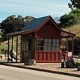# Gas station without pumps

## 2017 July 1

### Multiple-feedback bandpass active filter

Filed under: Robotics,Uncategorized — gasstationwithoutpumps @ 12:14
Tags: ,

I wanted to improve my understanding of active filters, beyond the very simple ones that I included in my textbook, so I decided to try implementing a bandpass filter using the multiple-feedback topology.  To make the design more concrete, I decided to make one that was centered at 2kHz, but firmly rejected 1.5kHz and 2.5kHz.Here is the design I came up with. The top part is the filter, while the bottom is just a reference voltage buffer. I’ll derive the values below.$I_1= (V_{in} -V_{m})/R_1$$I_2= (V_{ref}-V_{m})C_{2} s = (V_{o}-V_{ref})/R_2$$I_3= (V_{ref}-V_{m})/R_3$$I_4= (V_{o}-V_{m})C_{1}s$$I_1 + I_2 + I_3 +I_4 = 0$

We can simplify a bit, by replacing$V_{m}-V_{ref}$ with$-(V_{o}-V_{ref})/(\tau_2 s)$, where$\tau_2 = R_2 C_2$. Note that this corresponds to replacing the amplifier, R2, and C2 with an inverting high-pass filter.

It is conventional to recenter the voltages so that$V_{ref}$ is zero, which simplifies the algebra.  Doing that gives us$(V_{in}+V_{o}/(\tau_2 s))/R_1 + V_{o}/R_2 + V_{o}/(\tau_2 s R_3) + V_{o}(1+1/(\tau_2 s))C_{1}s=0$

Multiplying both sides by$\tau_2 s$ and rearranging gives us$-V_{in}\tau_2 s/R_1 = V_{o} \left(1/R_1 + \tau_2 s /R_2 + 1/R_3 + (\tau_2 s +1)(C_1 s)\right)$$\frac{V_{o}}{V_{in}} = -\tau_2 s / \left({1+ \tau_2 s R_1/R_2 + R_1/R_3 + (\tau_2 s +1)(R_1 C_1 s)}\right)$

More correctly, that should be$\frac{V_{o}-V_{ref}}{V_{in}-V_{ref}} = \ldots$, but I’ll just talk about the gain$G$.

Defining$\tau_1 = R_1 C_1$ we get$G = -\tau_2 s / \left(1+ R_1/R_3+ (\tau_2 R_1/R_2 + \tau_1) s + \tau_1\tau_2 s ^2)\right)$$G = -(\tau_2 s/R_1 )/ \left(1/R_1+1/R_3+ (\tau_2 /R_2 + \tau_1/R_1) s + \tau_1\tau_2 s ^2/R_1)\right)$

If we have$C_1=C_2=C$, which is a common design constraint, we can simplify to$G = -(R_2 C s/R_1 )/ \left(1/R_1+1/R_3+ 2 C s +C\tau_2 s ^2)\right)$$G = -(R_2 /R_1 )/ \left((1/R_1+1/R_3)(Cs)^{-1}+ 2 +\tau_2 s)\right)$

We can define$R_p = R_1 || R_3 = R_1R_3/(R_1+R_3)$ and$\tau_p = R_p C_1$.  Then we have$G = -(R_2 /R_1 )/ \left((\tau_p s)^{-1}+ 2 +\tau_2 s)\right)$

If we let$s = j \omega$, we get$G = -(R_2 /R_1 )/ \left(2 +j (\tau_2 \omega-(\tau_p \omega)^{-1})\right)$,

whose magnitude is maximized when$\tau_2 \omega-(\tau_p \omega)^{-1}=0$ or$\omega = 1/\sqrt{\tau_2\tau_p}$, and at that maximum the gain is$- R_2/ (2 R_1)$.

The corner frequencies, where the gain drops by the square-root of 2 occur when the real and imaginary parts of the denominator have the same magnitude, or$\tau_2 \omega-(\tau_p \omega)^{-1}= \pm 2$. If we take only the positive values of$\omega$, we get$\omega = \left(\pm 1 + \sqrt{(\tau_p+\tau_2)/\tau_p}\right) /\tau_2$, for a bandwidth of$2/\tau_2$, or$1/(\pi R_2 C_2)$ Hz.

For C=10nF and R2=220kΩ, we get a bandwidth of 144.7Hz.  With R1=6.8kΩ and R3=300Ω, Rp=287.32Ω, with time constant 2.8732 µs, giving a center frequency of 2001.8kHz.  I built this filter and tested it with the Analog Discovery 2:But when we look in detail at the peak, we can see that the center frequency is off a little and the gain isn’t nearly as big as it should be. I suspect that the problem is poor matching of the very cheap 10nF capacitors.

I also tried exciting the filter with wideband noise from the Analog Discovery 2, and doing an FFT of the input and output to get a different view of the response:The filter response looks a little larger taking the difference of FFTs (after averaging many FFTs, since each individual one has a lot of noise). Note the 60 Hz spike.

## 1 Comment »

1.[…] Multiple-feedback bandpass active filter I built a simple active filter to be part of a tone-detection circuit (looking for 2kHz). […]

Pingback by Digital tone detection with Goertzel’s algorithm | Gas station without pumps — 2017 July 9 @ 00:28

This site uses Akismet to reduce spam. Learn how your comment data is processed.